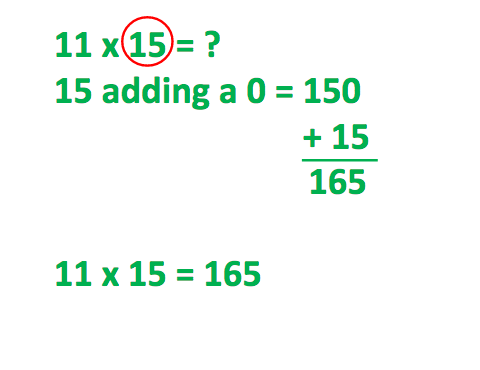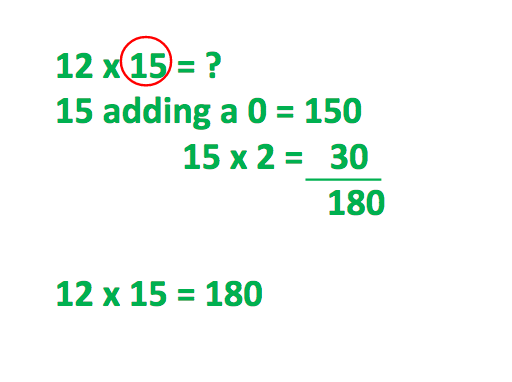Smartick is a fun way to learn math!Oct23

# Learn and Practice the 11 and 12 Times Tables

In today’s post, we’re going to talk about 11 and 12 times tables.

Schools generally teach the times tables from 1 to 10, and 11 and 12 times tables are something of an afterthought. However, the 11 and 12 times tables are gaining an increasing importance, and we’ll look at some strategies to help learn them today.

Some argue that there’s no need to learn multiplication tables after the number 10 in order to fit with a system of decimal learning but at Smartick we think that it is worth the extra effort to learn them.

To begin with, it’s obvious that the more techniques we have when it comes to operating, the faster we will be able to calculate in our minds.

In addition, the 12 times table helps us quickly work out calculations related to cultural values, such as dozens of objects (eggs, flowers…) measurements in feet and inches, or the number of months in a year. It can also help us when calculating the hours there are in a certain number of days since 24 (hours in a day) is 12 x 2.

Taking this into account, and since it wouldn’t make sense to move from the 10 times table to the 12 times table and skip out 11, we think that the benefits to our students much outweigh the effort spent to learn the 11 and 12 times tables.

So, with that in mind, we have some little tricks to help reduce that extra effort.

### Times tables: trick for the 11 times table

To multiply by 11, we add a zero to the number we’re going to multiply (in other words, we multiply by 10) and then we add the original number to the result.

Let’s look at an example:### Times tables: trick for the 12 times table

To multiply by 12, we add a zero to the number we’re going to multiply and then we add double the original number to the result.

Let’s look at an example:Here you can review some tricks for 1 to 10 times tables in a previous post.

We hope you’ve enjoyed today’s post, and that our tricks have helped you out.